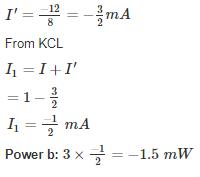Courses

# Analog Electronics - 6

## 10 Questions MCQ Test RRB JE for Electronics & Communication Engineering | Analog Electronics - 6

Description
This mock test of Analog Electronics - 6 for Electronics and Communication Engineering (ECE) helps you for every Electronics and Communication Engineering (ECE) entrance exam. This contains 10 Multiple Choice Questions for Electronics and Communication Engineering (ECE) Analog Electronics - 6 (mcq) to study with solutions a complete question bank. The solved questions answers in this Analog Electronics - 6 quiz give you a good mix of easy questions and tough questions. Electronics and Communication Engineering (ECE) students definitely take this Analog Electronics - 6 exercise for a better result in the exam. You can find other Analog Electronics - 6 extra questions, long questions & short questions for Electronics and Communication Engineering (ECE) on EduRev as well by searching above.
QUESTION: 1

### The current flowing through the 3 Ω resistor R1 is _____ A.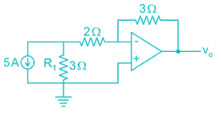Solution: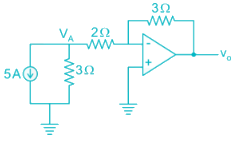Apply nodal at A: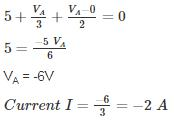Magnitude wise: 2 A

*Answer can only contain numeric values
QUESTION: 2

### An op-amp has a voltage gain of 100 dB at dc and a unity gain frequency of 5 MHz. The lower 3-dB cut off frequency is _______ Hz.

Solution:

100 dB voltage gain

20 log (Gain) = 100

Gain = 105

GBW = 5 MHz

Low frequency 3-dB =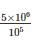= 50 Hz.

*Answer can only contain numeric values
QUESTION: 3

### An op-amp with slew rate 1 V/μ is used in the circuit below input step voltage Vi = V sin (105t) is given, the maximum value of V such that no raise time distortion occurs at the output is _____V.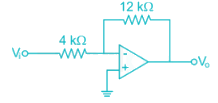Solution:

The output Vo = -3V sin (ω0t)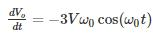For no distortion to occur

3Vω0 ≤ SR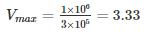*Answer can only contain numeric values
QUESTION: 4

The circuit shows an analog voltmeter of very high input impedance that uses an inexpensive moving coil-meter. The voltmeter measures the voltage ‘V’ applied between the op-amp’s positive-input terminal and ground. Assuming that the moving coil produces full-scale deflection when the current passing through it is 100 μA, the value of R (in kΩ) to obtain full-scale reading at +10 V is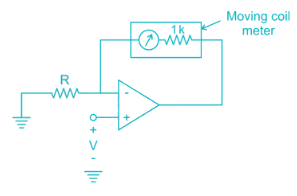Solution: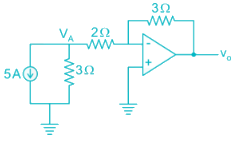VA = V (virtual ground)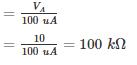QUESTION: 5

Circuit shows an op-amp circuit used for generating the square waveform.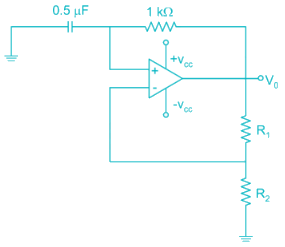If the output frequency required in 1 kHz. then the possible values of R1­ and R2 can be respectively

Solution:

Frequency of oscillation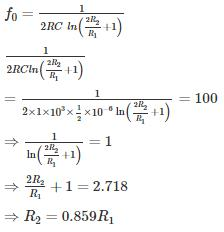If R1 = 2k

R2 = 0.859 × 2

= 1.7 k

Option 1 is correct.

*Answer can only contain numeric values
QUESTION: 6

The circuit shows the series voltage regulator.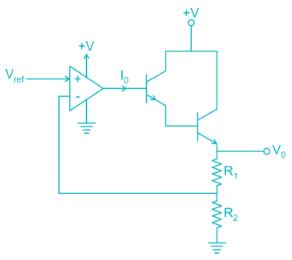If reference voltage Vref is 2V and R1 = 3K, R2 = 1K, β = 99 for both transistors. Then the value of current I0 in micro-amperes is _____ μA.

Solution:

V- = V+ = 2V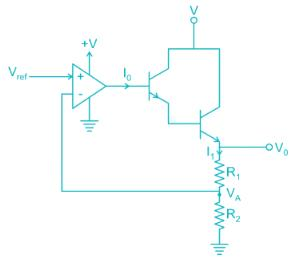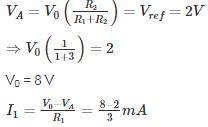= 2 mA

I1 = (1 + β) (1 + β) I0

2 mA = 104 I0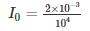I = 0.2 uA

QUESTION: 7

The circuit shows an – op-amp in Schmidt Trigger configuration. If the hysteresis width is 3V. Then the value of R1 and R2 is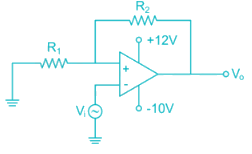Solution: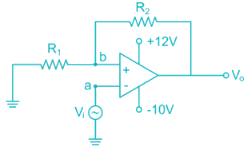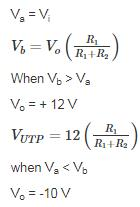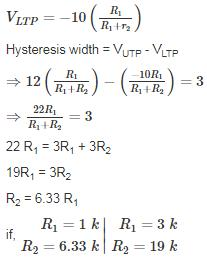*Answer can only contain numeric values
QUESTION: 8

For the amplifier circuit shown, the op-amp can deliver a maximum current of 100 mA and is powered by ±15 V supply. If the input to the amplifier is a triangular waveform as shown. The peak value of the output waveform is ______.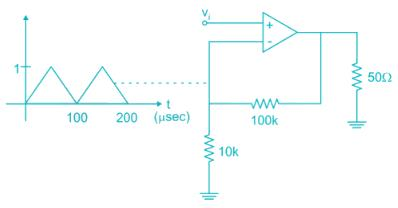Solution:

The circuit is non-inverting.

Amplifier: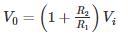= 11V

However the current flowing through load when V0 = 11 V is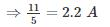Which is more than the current op-amp can supply.

Thus peak value is limited by the maximum current:

50 Ω ≪ 110 kΩ

All the current flows through 50 Ω resistor.

V0 = (100 mA) (50 Ω)

= 5 V

QUESTION: 9

An op-amp circuit is shown in the figure. If Vin is an AC source of frequency ω.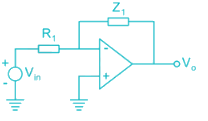Consider the following statements:
a) For ω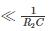Circuit is an integrator
(b) For ω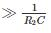circuit is an Amplifier

Solution: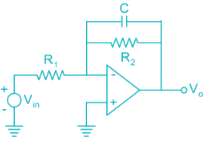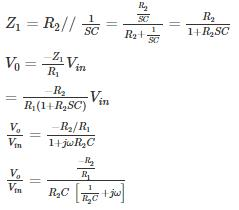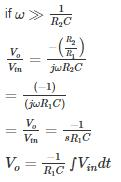Circuit acts like an Integrator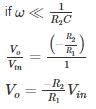Circuit acts an Inverting Amplifier

The given conditions in question is opposite Hence None of a and b is correct

*Answer can only contain numeric values
QUESTION: 10

The average power delivered by the 3 V source is ________ mW.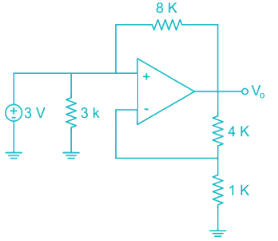Solution: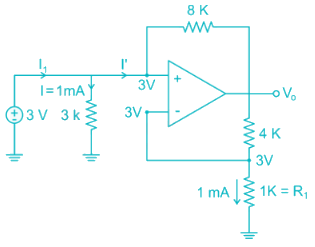V- = V+ = 3 V [Virtual ground]

Current through 1 k resistor

I = 1 mA

Voltage across 1k resistor from voltage division

Voltage across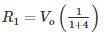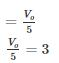Vo = 15 V

KVL at V+ node:

3 – I'(8) = Vo

3 – I'(8) = 15

3 – 15 = I'(8)